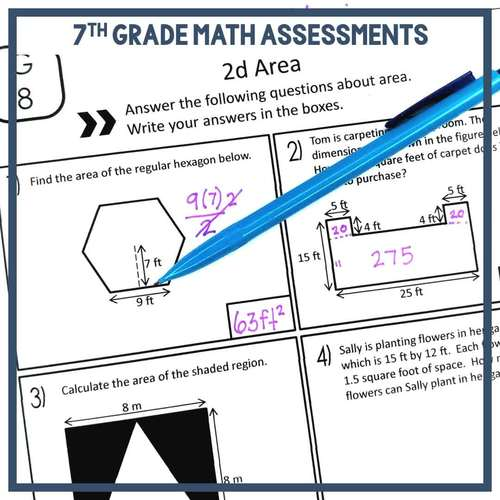# 7th Grade Math Assessments Common Core Bundle EDITABLE | 7th Grade Math Tests

Rated 4.87 out of 5, based on 44 reviews
44 Ratings;
7th, Homeschool
Subjects
Resource Type
Standards
Formats Included
• Zip
Pages
65 pages
\$12.00
List Price:
\$18.50
You Save:
\$6.50
Bundle
\$12.00
List Price:
\$18.50
You Save:
\$6.50
Bundle
Report this resource to TPT

#### Products in this Bundle (7)

showing 1-5 of 7 products

##### Also included in
1. Looking to save time, money and sanity? Then this 7th grade math curriculum and activities bundle is for you! This curriculum is aligned to the 7th grade common core math standards. Each unit includes: guided notes, assessments/worksheets and activities such as: task cards, stations, mystery pictur
Price \$250.00Original Price \$403.00Save \$153.00

### Description

Save yourself some time with these EDITABLE 7th grade math assessments. These math assessments are aligned to the 7th grade math common core. Focus is placed on fluency AND clear understanding of concepts. Students will calculate, write, model, analyze and explain to show their understanding of the common core concepts.

30 assessments covering rational numbers, expressions, equations, inequalities, proportional reasoning, proportional relationships, geometry, statistics, and probability.

By purchasing this Bundle you are saving over 30% off each individual product.

This product is EDITABLE!

• Includes a complete PDF document of assessments
• Includes a PowerPoint document that allows you to edit the questions
• You can create various versions to use in your math classroom

• Quick assessments
• Quizzes
• Reviews
• Interventions
• Homework
• Track student progress and mastery of concepts

Assessments are specifically aligned to 7th Grade CCSS

Rational Numbers

• Integer addition and subtraction fluency
• Integer multiplication and division fluency
• Integer addition and subtraction with number lines
• Rational number addition and subtraction fluency
• Rational number multiplication and division fluency
• Rational number understanding
• Rational number word problems

Algebra: Expressions, Equations & Inequalities

• Simplifying expressions fluency
• Applied expressions
• Solving equations fluency
• Word problems and equations
• Solving inequalities fluency
• Word problems and inequalities

Proportional Relationships

• Calculating Unit Rates
• Proportional Relationships
• Constant of Proportionality
• Proportional Graphs and Equations

Proportional Reasoning

• Ratios and Percent Problems
• Scale Drawings

Geometry

• Angle Basics
• Angles & Algebra
• Triangle Construction
• Circles: Circumference and Area Fluency
• Circles: Circumference and Area Understanding
• 2d Area
• 3d Surface Area and Volume
• 3d Slicing

Probability

• Probability
• Compound Events

Statistics

• Statistics

Also available for 8th Grade Math

"I am so thankful to have come across these! Well done and saves me a lot of time!"

"I am so glad I purchased this resource! The items included in this will be perfect to check for iep goals and make sure my kiddos are reaching proficiency in particular standards as well as to check in for retention. Thanks for creating this product!"

FOLLOW ME for more great products to make sense of math!

Remember that leaving feedback earns you points toward FREE TPT purchases!

Please feel free to contact me with any questions!

Michelle,

Make Sense of Math

Total Pages
65 pages
Included
Teaching Duration
N/A
Report this resource to TPT
Reported resources will be reviewed by our team. Report this resource to let us know if this resource violates TPT’s content guidelines.

### Standards

to see state-specific standards (only available in the US).
Solve problems involving scale drawings of geometric figures, including computing actual lengths and areas from a scale drawing and reproducing a scale drawing at a different scale.
Draw (freehand, with ruler and protractor, and with technology) geometric shapes with given conditions. Focus on constructing triangles from three measures of angles or sides, noticing when the conditions determine a unique triangle, more than one triangle, or no triangle.
Describe the two-dimensional figures that result from slicing three-dimensional figures, as in plane sections of right rectangular prisms and right rectangular pyramids.
Know the formulas for the area and circumference of a circle and use them to solve problems; give an informal derivation of the relationship between the circumference and area of a circle.
Use facts about supplementary, complementary, vertical, and adjacent angles in a multi-step problem to write and solve simple equations for an unknown angle in a figure.